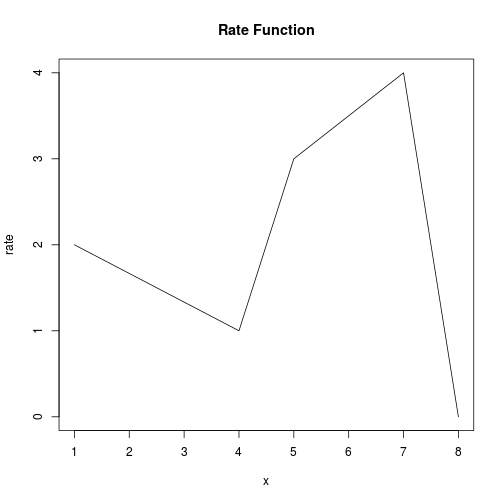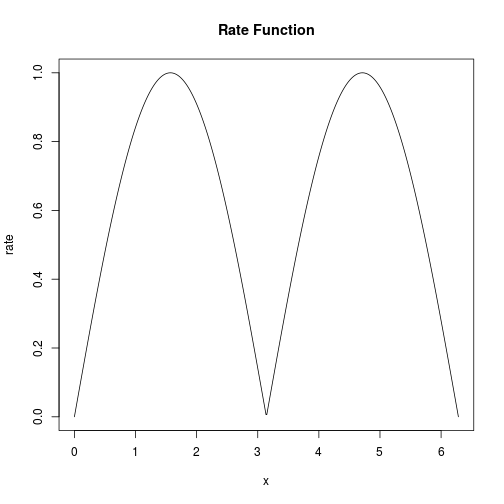This document provides a brief introduction into the R package IPPP with an overview of the available functions and some theoretical background.

## Introduction

A number of problems can be modeled as points (“events”) that are random, (stochastically) independent of each other, and occur with a changing rate on a line. This line might be time or a spatial dimension. Examples of this are animal sightings in time or in space, or the times at which customers enter a supermarket. The mathematical structure describing these processes is called an inhomogeneous Poisson point process, here abbreviated as IPPP. The package IPPP provides methods to

1. Simulate the number and location of points in IPPPs
2. Determine probability density functions for the location of points

with the option to include information that is available upfront into the analysis. This information can for example be

• the total number of points occurring or
• the a priori known location of points

## Rate Functions

An IPPP is defined by a positive function determining the rate with which points occur. This function is called the rate function. The expected number of points between some values a and b is given as the integral from a to b over the rate function.

For the package, rate functions are always assumed to be piecewise linear. This makes it possible to define them using two vectors, one with the x values (named `xrate` ) and one with the corresponding y values (named `yrate` ). The basic way to define a rate function is to define these vectors by entering them by hand.

``````xrate=c(1,4,5,7,8)
yrate=c(2,1,3,4,0)
plot(xrate,yrate,type='l',ylab='rate',xlab='x',main='Rate Function' )
``````A more convenient way is to take an already existing function (here the absolute value of sine) and approximate it with a suitable precision by evaluating it at a vector `xrate`

``````#rate function to approximate
ratefunction=function (x){
return(abs(sin(x)))
}
#where to approximate the rate function
xrate=seq(0,2*pi,length.out = 500)
#approximate
yrate=ratefunction(xrate)
plot(xrate,yrate,type='l',ylab='rate',xlab='x',main='Rate Function' )
``````The vector `xrate` should be chosen according to the irregularity of the rate function; the more irregular the rate function is, the finer `xrate` needs to be.[The error of this approximation can be determined directly, e.g. the difference in the expected number of points is the integral over the the difference between the exact rate function and its approximation.]

## Features

The package allows to simulate IPPPs and generate their probability density functions. The application can be subdivided into the cases where

1. No additional information is available
2. The number of points occurring is known, and their location is of interest
3. The location of one point is known, and the location of other points relative to this point is of interest

### No Additional Information Available: Determine Location and Number of Points

In the case where no additional information is available, the functions

• `IPPPuncond` simulates where and how many points occur
• `IPPPinterval` simulates how many points occur in an interval

### Number of Points Known: Determine Their Location and Densities

If the number of points occurring is known, the functions

• `IPPPcond` simulates where these points occur
• `IPPPnthpointdens` determines the probability density of the points

### One Location Known: Determine Location and Densities of Points Above/Below

If the location of one point is already known, the functions

• `IPPPconddens` determines the probability density function for the location of the n-th point above/below the known point for arbitrary n.
• `IPPPcondrandno` generates random numbers that correspond to the location of the n-th point above/below the known point

## Examples

For examples please refer to the help pages of the corresponding functions

## References

Hohmann, Niklas. “Conditional Densities and Simulations of Inhomogeneous Poisson Point Processes: The R package "IPPP”“ arXiv 2019. https://arxiv.org/abs/1901.10754 .